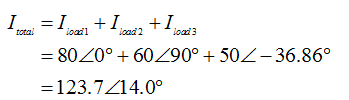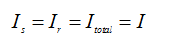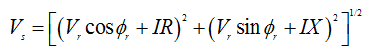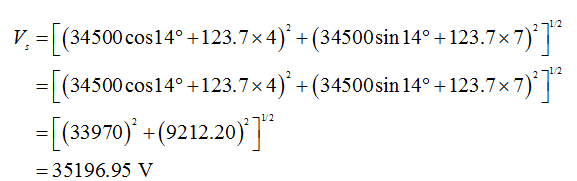# The following three-phase loads are connected to a short transmission line with impedance per wire of 4 + j7 ohms: Load 1: Resistive load drawing 80 A, Load 2: Capacitor drawing 60 A, Load 3: Inductive load drawing 50 A at 80 %          power factor. What is the sending end      power factor for a receiving end voltage of 34.5 kV?         a. 96 % b. 97 % c. 98 % d. 99 %

Question
1 views
1. The following three-phase loads are connected to a short transmission line with impedance per wire of 4 + j7 ohms: Load 1: Resistive load drawing 80 A, Load 2: Capacitor drawing 60 A, Load 3: Inductive load drawing 50 A at 80 %          power factor. What is the sending end      power factor for a receiving end voltage of 34.5 kV?

a. 96 % b. 97 % c. 98 % d. 99 %

check_circle

Step 1

• The power dissipated by the resistive load will be purely active because the power factor is unity.
• There will be reactive power injected by the capacitor.
• Active and reactive power drawn by the inductive load.
Step 2Step 3

For the short transmission line, the relation between the current is,The sending end voltage is given by the following expression,Substitute Z as the transmission line impedance....

### Want to see the full answer?

See Solution

#### Want to see this answer and more?

Solutions are written by subject experts who are available 24/7. Questions are typically answered within 1 hour.*

See Solution
*Response times may vary by subject and question.
Tagged in